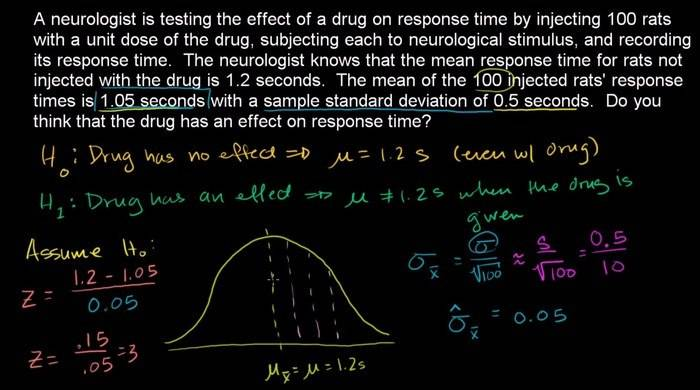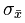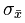# Rejecting a null hypothesis without a z-score or p-value?

• I
Gold MemberIs it possible to reject the null hypothesis without calculating the z-score? We estimated theto be 0.05
—>2*= 0.1; and 2σ = 95% (empirical rule)
Therefore there is a 95% confidence interval between 0.95 (=1.05 - 0.1) and 1.15 (=1.05+0.1)

So we can say we are 95% confident that the μx (of the drug administered rats) is between 0.95 and 1.15. Therefore we can reject the hypothesis because μ of the non-drug-rats is 1.2, which falls outside of the 95%.

Is this reasoning correct?

Gold Member
Okay, my professor said it's in correct in a sense, but I need to be careful about notations. He explained why, but I'm downloading LaTeX to see his notations. I'll update this thread to avoid confusion asap.

Merlin3189
Homework Helper
Gold Member
Are you not simply calculating Z>2 ∴ p<0.05 rather than calculating the more accurate Z =3 and P=0.003 ?

Gold Member
Not really. I'm not calculating the Z-score directly. I'm only interpreting the results with the empirical rule and the confidence interval; so I wondered if you can reject a null hypothesis without mentioning the z-scores.

Merlin3189
Homework Helper
Gold Member
—>2*= 0.1; and 2σ = 95% (empirical rule) Isn't this just remembering that p=0.025 for z=2 ?
Therefore there is a 95% confidence interval between 0.95 (=1.05 - 0.1) and 1.15 (=1.05+0.1) and this is saying Z=2 for sample means outside 0.95 - 1.15, therefore that is the 95% confidence range?
I'm not saying you're doing anything wrong, simply that you are doing what people do a Z test. Many people do use a simplified table that has only Z values for 95%, 97.5%, 99%, 99.5%, 99.9%, 99.95% say. You're just memorising the one value that interests you (95%) and checking that Z is greater than 2. This is fine, especially if you were working to 99.95% or better. Sometimes you may want to calculate p more precisely to give you a closer estimate of confidence: perhaps you'd be happier if your result came out at 99% rather than 96%. It doesn't look much, but the risk of error is 4x greater at 96% than 99%. (Although many people take 95% as near enough certain, I could argue that it is very dubious in some circumstances.)

•CynicusRex
Therefore there is a 95% confidence interval between 0.95 (=1.05 - 0.1) and 1.15 (=1.05+0.1)

So we can say we are 95% confident that the μx (of the drug administered rats) is between 0.95 and 1.15. Therefore we can reject the hypothesis because μ of the non-drug-rats is 1.2, which falls outside of the 95%.

Is this reasoning correct?

Using a confidence interval to reject a null hypothesis is excessively conservative, so it really isn't what you want to do. Moreover, your interpretation of the confidence interval is incorrect. It doesn't say anything about the probability or confidence about the location of the mean (I can easily construct a 95% confidence interval that has zero probability of containing the mean).

Why don't you want to calculate the z-score? You have all of the information you need in order to do it.

Gold Member
I was merely wondering if you can reject a null without mentioning a z-score. It has nothing to do with not wanting to do it. It's a question to make sure I'm not confusing things.

chiro